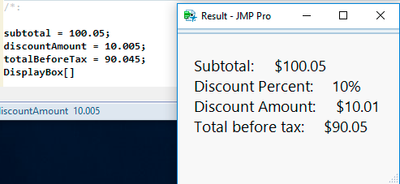Our World Statistics Day conversations have been a great reminder of how much statistics can inform our lives. Do you have an example of how statistics has made a difference in your life? Share your story with the Community!
HighlightedPhil_Brown
Super User

## How to handle rounding error

I have this script which does a simple calculation and displays result in a window:

subtotal = 100.05;

discountPercent = 0.1;

discountAmount = subtotal * discountPercent;

totalBeforeTax = subtotal - discountAmount;

formattedMessage = Concat(

"Subtotal:",Repeat(" ",5), Format(subtotal, "Currency"),  "\!n",

"Discount Percent:", Repeat(" ",5), Format(discountPercent, "Percent"), "\!n",

"Discount Amount:", Repeat(" ",5), Format(discountAmount, "Currency"), "\!n",

"Total before tax:", Repeat(" ",5), Format(totalBeforeTax, "Currency"), "\!n"

);

formattedMessageDisplay = New Window( "Result",

Lineup Box( N Col( 1 ), Spacing( 0, 0 ), Border Box( Left( 20 ), Right( 20 ), top( 20 ), bottom( 20 ),

Text Box( formattedMessage, <<SetFontSize( 14 ) ) )

),

Show(subtotal, discountAmount, totalBeforeTax)

);

It produces the following, in the log and the output window :So using Format(totalBeforeTax, "Currency"), results in totalBeforeTax being "rounded up". I can think of a variety of ways of handling this, but wondered what would be most recommended.

PDB
1 ACCEPTED SOLUTION

Accepted Solutions
Highlighted

## Re: How to handle rounding error

You might want to round/floor/ceiling the discountAmount calculation.  Round has a second argument that you might want to set to 2 for digits after the decimal.  Floor and ceiling will need something like floor(x*100)/100 to get a similar result.

subtotal = 100.05;

discountPercent = 0.1;

discountAmount = round(subtotal * discountPercent,2);

totalBeforeTax = subtotal - discountAmount;

show(discountAmount, totalBeforeTax);

discountAmount = 10.01;

totalBeforeTax = 90.04;

The format function in your original code is on the knife-edge of rounding up or down with the 0.005 rounded to two places.  The discrepancy in the presentation is triggered by the value having a minus in one case and a plus in the other...both values are rounded up, but one of them has negative discountAmount in the calculation.

Floating point numbers used in JMP represent numbers like 0.01 as a truncated repeating binary fraction.  Just like 1/3 isn't exactly 0.333333333333, the internal representation isn't exact (except for binary fractions like 1/2, 1/4, 1/8, ...).  if you add up 100 pennies, represented as 0.01, you'll get a number that is very close to 1.00, but different in the 16'th decimal place.  This very tiny error has always made accounting packages choose another representation: java - Why not use Double or Float to represent currency? - Stack Overflow

total = 0;

For( i = 1, i <= 100, i++, total += .01 );

show(  format(total,"fixed",20,16) );

Format(total, "fixed", 20, 16) = "1.0000000000000007";

Craige
6 REPLIES 6
Highlighted

## Re: How to handle rounding error

I don't do a lot of financial reporting, however, when I need to worry about rounding errors, I just use the functions Round(),Ceiling() and Floor().

Jim
HighlightedPhil_Brown
Super User

## Re: How to handle rounding error

Hi Jim,

Thanks for you reply. So, Floor and Ceiling round to the nearest integer. Format (x, "Currency") basically appears to use Round(x,2). One would think the "Currency" format would employ a truncation method rather than rounding.

PDB
Highlighted

## Re: How to handle rounding error

You might want to round/floor/ceiling the discountAmount calculation.  Round has a second argument that you might want to set to 2 for digits after the decimal.  Floor and ceiling will need something like floor(x*100)/100 to get a similar result.

subtotal = 100.05;

discountPercent = 0.1;

discountAmount = round(subtotal * discountPercent,2);

totalBeforeTax = subtotal - discountAmount;

show(discountAmount, totalBeforeTax);

discountAmount = 10.01;

totalBeforeTax = 90.04;

The format function in your original code is on the knife-edge of rounding up or down with the 0.005 rounded to two places.  The discrepancy in the presentation is triggered by the value having a minus in one case and a plus in the other...both values are rounded up, but one of them has negative discountAmount in the calculation.

Floating point numbers used in JMP represent numbers like 0.01 as a truncated repeating binary fraction.  Just like 1/3 isn't exactly 0.333333333333, the internal representation isn't exact (except for binary fractions like 1/2, 1/4, 1/8, ...).  if you add up 100 pennies, represented as 0.01, you'll get a number that is very close to 1.00, but different in the 16'th decimal place.  This very tiny error has always made accounting packages choose another representation: java - Why not use Double or Float to represent currency? - Stack Overflow

total = 0;

For( i = 1, i <= 100, i++, total += .01 );

show(  format(total,"fixed",20,16) );

Format(total, "fixed", 20, 16) = "1.0000000000000007";

Craige
HighlightedPhil_Brown
Super User

## Re: How to handle rounding error

Thanks Craige,  indeed I've been considering building an interface to allow JMP to access java's BigDecimal class. Are there any plans to include this as a type in the next version of JMP?

PDB
HighlightedPhil_Brown
Super User

## Re: How to handle rounding error

Another thing I thought of was to convert to string and use Regex.

PDB
Highlighted

## Re: How to handle rounding error

No Big Number plans at this time.  There were a lot of interesting points being made in the StackOverflow link.  Different solutions might be appropriate depending on what you'll do with the numbers.

Craige

Craige
Article Labels

There are no labels assigned to this post.